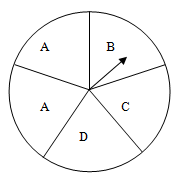Study Materials: ncert solutions

Our ncert solutions for Exercise 5.3 Class 8 maths 5. Data Handling - ncert solutions - Toppers Study is the best material for English Medium students cbse board and other state boards students.

Solutions ⇒ Class 8th ⇒ Mathematics ⇒ 5. Data Handling

# Exercise 5.3 Class 8 maths 5. Data Handling - ncert solutions - Toppers Study

Topper Study classes prepares ncert solutions on practical base problems and comes out with the best result that helps the students and teachers as well as tutors and so many ecademic coaching classes that they need in practical life. Our ncert solutions for Exercise 5.3 Class 8 maths 5. Data Handling - ncert solutions - Toppers Study is the best material for English Medium students cbse board and other state boards students.

## Exercise 5.3 Class 8 maths 5. Data Handling - ncert solutions - Toppers Study

CBSE board students who preparing for class 8 ncert solutions maths and Mathematics solved exercise chapter 5. Data Handling available and this helps in upcoming exams 2023-2024.

### You can Find Mathematics solution Class 8 Chapter 5. Data Handling

• All Chapter review quick revision notes for chapter 5. Data Handling Class 8
• NCERT Solutions And Textual questions Answers Class 8 Mathematics
• Extra NCERT Book questions Answers Class 8 Mathematics
• Importatnt key points with additional Assignment and questions bank solved.

NCERT Solutions do not only help you to cover your syllabus but also will give to textual support in exams 2023-2024 to complete exercise 5.3 maths class 8 chapter 5 in english medium. So revise and practice these all cbse study materials like class 8 maths chapter 5.3 in english ncert book. Also ensure to repractice all syllabus within time or before board exams for ncert class 8 maths ex 5.3 in english.

See all solutions for class 8 maths chapter 5 exercise 5 in english medium solved questions with answers.

### Exercise 5.3 class 8 Mathematics Chapter 5. Data Handling

• Exercise 5.3 Class 8 Maths 5. Data Handling - Ncert Solutions - Toppers Study
• Class 8 Ncert Solutions
• Solution Chapter 5. Data Handling Class 8
• Solutions Class 8
• Chapter 5. Data Handling Exercise 5.3 Class 8

## Exercise 5.3 Class 8 maths 5. Data Handling - ncert solutions - Toppers Study

Exercise 5.3

1. List the outcomes you can see in these experiments.

Spinning a wheel

Tossing two coins togetherOn spinning the given wheel, the possible outcomes are A, B, C, and D.

By tossing two coins together , the possible outcomes ate HT, TH, HH, TT, where H and T presents head and tail of coins respectively.

2. When a die is thrown

1.  list the  possible outcomes of an event of getting
1. (a) a prime number (b) not a prime number
2. (a) a number greater than 5 (b) a number not greater than 5
3. (a) When a dice is thrown, the possible outcomes, 2, 3, 5, are prime numbers. Hence, these are the possible outcomes of an event of not getting a prime number on the face of a dice

(b) Out of these outcomes 1, 4, 6 are not prime numbers. Hence these are the outcomes of           an event of not getting a prime number on the face of a dice.

(ii)  (A) out of these outcomes, a number greater than 5 is possible 6 when the number of face of

The dice.

(b)  Out of these outcomes, a number is not greater than 5 is possible when the number the second

Number on the face of the dice is anyone of the outcomes 1, 2, 3, 4, 5.

##### Other Pages of this Chapter: 5. Data Handling

Important Study materials for classes 06, 07, 08,09,10, 11 and 12. Like CBSE Notes, Notes for Science, Notes for maths, Notes for Social Science, Notes for Accountancy, Notes for Economics, Notes for political Science, Noes for History, Notes For Bussiness Study, Physical Educations, Sample Papers, Test Papers, Mock Test Papers, Support Materials and Books.Mathematics Class - 11th

NCERT Maths book for CBSE Students.

books

## Study Materials List:

##### Solutions ⇒ Class 8th ⇒ Mathematics
1. Rational Numbers
2. Linear Equations in One Variable
4. Practical Geometry
5. Data Handling
6. Squares and Square Roots
7. Cubes and Cube Roots
8. Comparing Quantities
9. Algebraic Expressions and Identities
10. Visualising Solid Shapes
11. Mensuration
12. Exponents and Powers
13. Direct and Inverse Proportions
14. Factorisation
15. Introduction to Graphs
16. Playing with Numbers

New Books